Courses

# Solutions of Electricity (Page No- 47) - Physics By Lakhmir Singh, Class 10 Class 10 Notes | EduRev

## Class 10 : Solutions of Electricity (Page No- 47) - Physics By Lakhmir Singh, Class 10 Class 10 Notes | EduRev

The document Solutions of Electricity (Page No- 47) - Physics By Lakhmir Singh, Class 10 Class 10 Notes | EduRev is a part of the Class 10 Course Lakhmir Singh & Manjit Kaur Solutions: Class 10 Science.
All you need of Class 10 at this link: Class 10

Page No:47

Question 1:
Are the lights in your house wired in series ?
Solution :
No, they are wired in parallel.

Question 2:
What happens to the other bulbs in a series circuit if one bulb blows off ?
Solution :
All the other bulbs also stop glowing.

Question 3:
What happens to the other bulbs in a parallel circuit if one bulb blows off ?

Solution :
All the other bulbs keep glowing.

Question 4:
Which type of circuit, series or parallel, is preferred while connecting a large number of bulbs :
(a) for decorating a hotel building from outside ?
(b) for lighting inside the rooms of the hotel ?

Solution :
(a) Series
(b) Parallel

Question 5:
Draw a circuit diagram to show how two 4 V electric lamps can be lit brightly from two 2 V cells.
Solution :
The two lamps (of 4V each) should be arranged in parallel with the two 2V cells.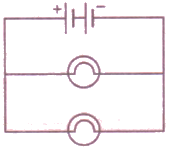Question 6:
Why is a series arrangement not used for connecting domestic electrical appliances in a circuit ?
Solution :
A series arrangement is not used for connecting domestic electrical appliances in a circuit because if one electrical appliance stops working due to some defect, then all other appliance also stop working as the whole circuit is broken.

Question 7:
Give three reasons why different electrical appliances in a domestic circuit are connected in parallel.
Solution :
Different electrical appliances in a domestic circuit are connected in parallel because of the following advantages:
(i) If one electrical appliance stops working due to some defect, then all other appliances keep working properly.
(ii) Each electrical appliance has its own switch due to which it can be turned on or turned off independently, without affecting other appliances.
(iii) Each electrical appliance gets the same voltage as that of the power supply line.

Question 8:
Ten bulbs are connected in a series circuit to a power supply line. Ten identical bulbs are connected in a
(a) parallel circuit to an identical power supply line.
(b) Which circuit would have the highest voltage across each bulb ?
(c) In which circuit would the bulbs be brighter ?
(d) In which circuit, if one bulb blows out, all others will stop glowing ?
(e) Which circuit would have less current in it ?

Solution :
(a) Parallel circuit
(b) Parallel circuit
(c) Series circuit
(d) Series circuit.

Question 9:
Consider the circuits given below :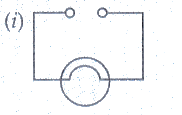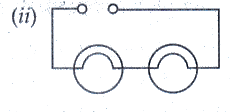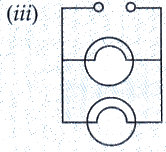(a) In which circuit are the lamps dimmest ?
(b) In which circuit or circuits are the lamps of equal brightness to the lamps in circuit (/) ?
(c) Which circuit gives out the maximum light ?

Solution :
(a) circuit (ii)
(b) circuit (iii)
(c) circuit (iii)

Page No:48

Question 10:
If you were going to connect two light bulbs to one battery, would you use a series or a parallel arrangement ? Why ? Which arrangement takes more current from the battery ?
Solution :
Parallel arrangement because if one electrical bulb stops glowing due to some defect the other will keep glowing.
Parallel arrangement takes more current from the battery due to its lesser equivalent resistance.

Question 11:
(a) Which is the better way to connect lights and other electrical appliances in domestic wiring : series circuits or parallel circuits ? Why ?
(b) Christmas tree lamps are usually wired in series. What happens if one lamp breaks ?
(c) An electrician has wired a house in such a way that if a lamp gets fused in one room of the house, all the lamps in other rooms of the house stop working. What is the defect in the wiring ?
(d) Draw a circuit diagram showing two electric lamps connected in parallel together with a cell and a switch that works both lamps. Mark an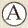on your diagram to show where an ammeter should be placed to measure the current.

Solution :
(a) Parallel circuits – Because if one electrical appliance stops working due to some defect, then all other appliances in the circuit will keep working properly.
(b) All the other lamps stop glowing.
(c) All lamps are connected in series.
(d)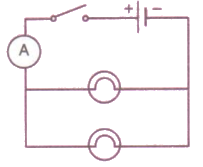Question 14:
(a) Draw a circuit diagram showing two lamps, one cell and a switch connected in series.
(b) How can you change the brightness of the lamps ?

Solution :

(a)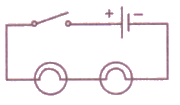(b) The brightness of the lamps can be changed by connecting the lamps in parallel.

Question 15:
Consider the circuit given below where A, B and C are three identical light bulbs of constant resistance.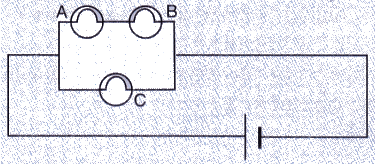(a) List the bulbs in order of increasing brightness.
(b) If C burns out, what will be the brightness of A now compared with before ?
(c) If B burns out instead, what will be the brightness of A and C compared with before ?

Solution :
(a) C will be the brightest. Voltage will be distributed equally between A and B, so they will have equal brightness but lesser than that of C.
(b) A gets the same voltage as before, so its brightness remains the same.
(c) If B burns put, A will also stop glowing because it is connected in series with B. However, brightness of C remains the same.

Question 16:
How do you think the brightness of two lamps arranged in parallel compares with the brightness of two lamps arranged in series (both arrangements having one cell) ?
Solution :
The brightness of two lamps arranged in parallel is much more those arranged in series.

Question 17:
If current flows through two lamps arranged :
(a) in series,
(b) in parallel,
and the filament of one lamps breaks, what happens to the other lamp ? Explain your answer.

Solution :
(a) In case of series connection, if filament of one lamp breaks, the other wil stop glowing.
(b) In case of parallel connection, if filament of one lamp breaks, the other will keep glowing.

Page No:49

Question 18:
The figure below shows a variable resistor in a dimmer switch.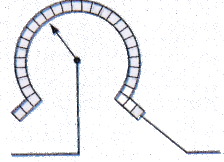How would you turn the switch to make the lights : (a) brighter, and (b) dimmer ? Explain your answer.
Solution :
(a) Turn the switch to right side so as the resistance decreases.
(b) Turn the switch to the left side so as the resistace increases.

Page No:58

Question 1:
State two factors on which the electrical energy consumed by an electrical appliance depends.
Solution :
Electrical energy consumed by an electrical appliance depends on:
1. Power rating of the appliance.
2. Time for which the appliance is used.

Question 2:
Which one has a higher electrical resistance : a 100 watt bulb or a 60 watt bulb ?
Solution :
60 watt bulb, because power is inversely proportional to the resistance.

Question 3:
Name the commercial unit of electric energy.
Solution :
Kilowatt-hour is the commercial unit of electric energy.

Question 4:
An electric bulb is rated at 220 V, 100 W. What is its resistance ?
Solution :
V = 220 V, P = 100W
R=?
We know that
P = V2/R
Thus
R = V2/P = 2202/100 = 484ohm

Question 5:
What is the SI unit of (i) electric energy, and (ii) electric power ?
Solution :
(i) joule
(ii) watt

Question 6:
Name the quantity whose unit is (i) kilowatt, and (ii) kilowatt-hour.
Solution :
(i) Electric power
(ii) Electric energy

Question 7:
Which quantity has the unit of watt ?
Solution :
Electric power has the unit of watt.

Question 8:
What is the meaning of the symbol kWh ? Which quantity does it represent ?
Solution :
kWh is the short form of kilowatt-hour, which is the commercial unit of electrical energy.

Question 9:
If the potential difference between the end of a wire of fixed resistance is doubled, by how much does the electric power increase ?
Solution :
P = V2/R
R is fixed.
V becomes double.
Now, P = (2V)2/R = 4 V2/R
So, the electric power becomes four times its previous value.

Question 10:
An electric lamp is labelled 12 V, 36 W. This indicates that it should be used with a 12 V supply. What other information does the label provide ?
Solution :

Other information is that it will consume energy at the rate of 36 J/s.

Question 11:
What current will be taken by a 920 W appliance if the supply voltage is 230 V ?
Solution :
P = 920W, V = 230V, I = ?
We know that
P = V x I,
920 = 230 x I
I = 920/230 = 4amp

Question 12:
Define watt. Write down an equation linking watts, volts and amperes.
Solution :
When an electrical appliance consumes electrical energy at the rate of 1 joule per second, its power is said to be 1 watt.
1 watt = 1 volt x 1 ampere.

Question 13:
Define watt-hour. How many joules are equal to 1 watt-hour ?
Solution :
One watt hour is the amount of electrical energy consumed when an electrical appliance of 1 watt power is used for 1 hour.
1 watt hour = 3600 joules

Question 14:
How much energy is consumed when a current of 5 amperes flows through the filament (or element) of a heater having resistance of 100 ohms for two hours ? Express it in joules.
Solution :
I = 5amp, R = 100ohms, t = 2h
We know that
Electric energy consumed = P x t = I*I*Rt
= 25 x 100 x 2
= 5000 Wh
= 5 kwh
We know that 1kwh = 3.6 x 106 J
Therefore, 5kwh = 5 x 3.6 x 106 J = 18 x 106 J.

Question 15:
An electric bulb is connected to a 220 V power supply line. If the bulb draws a current of 0.5 A, calculate the power of the bulb.
Solution :
V = 220V, I = 0.5amp, P = ?
We know that
P = VI = 220X0.5
P =110 watt.

Question 16:
In which of the following cases more electrical energy is consumed per hour ?
(i) A current of 1 ampere passed through a resistance of 300 ohms.
(ii) A current of 2 amperes passed through a resistance of 100 ohms.

Solution :

(i) R = 300 ohm. I = 1 A. t = 1h
P = I2R = 12 x 300 = 300 W
E= Pxt= 300 x 1 = 300 Wh

(ii) R = 100 ohm. I = 2 A. t = 1h
P = I2R = 22 x 100 = 400 W
E = Pxt = 400 x 1 = 400 Wh
Hence. in case (ii). the electrical energy consumed per hour is more.

Question 17:
An electric kettle rated at 220 V, 2.2 kW, works for 3 hours. Find the energy consumed and the current drawn.
Solution :
V = 220V, P = 2.2kW = 2200W, t = 3h
We know that
Electrical energy consumed = Pxt = 2.2×3 = 6.6 kWh
We have, P = V x I
2200 = 220 x I
I = 10amp

Page No:59

Question 18:
In a house two 60 W electric bulbs are lighted for 4 hours, and three 100 W bulbs for 5 hours everyday. Calculate the electric energy consumed in 30 days.

Solution :

Case 1:
Power, P1 = 60W
Number, t1 = 2
Time for use, t1 = 4h everyday
Electrical energy consumed everyday, E1 = n1 x P1 x t1 = 2 x 60 x 4 = 480 Wh = 0.48kWh
Electrical energy consumed in 30 days = 30 x 0.48 = 14.4kWh

Case 2:
Power, P2 = 100W
Number, n2 = 3
Time for use, t2 = 5h everyday
Electrical energy consumed everyday, E2 = n2 x P2 x t2
= 3x 100 x 5 = 1500 = 1.5kWh
Electrical energy consumed in 30 days = 30x 1.5.45kWh
Total electrical energy consumed in 30 days = 14.4kWh + 45kWh = 59.4kWh

Question 19:
A bulb is rated as 250 V; 0.4 A. Find its : (i) power, and (ii) resistance.

Solution :

V = 250VJ = 0Aarnp
(i) We k now that
Power = VI =250X0.4 = 100watt
(ii) We have
P = I2R
100 = 0.42XR
R = 625ohm

Question 20:
For a heater rated at 4 kW and 220 V, calculate :
(a) the current,
(b) the resistance of the heater,
(c) the energy consumed in 2 hours, and
(d) the cost if 1 kWh is priced at ₹ 60.
Solution :

Given

P = 4kw. V = 220v

(a) 1=?

Power = VI = 250X1

4000 = 2501

I = 16amp

(b) R = ?

p = 12R

P=162XR

R = 4000/162

R = 15.25ohm

(c) Energy consumed in two hour=PXt

= 4X2

= 8kw-hr

(d) If lkwh = Rs 4.6

total cost = 8 x 4.6 = Rs 36.8

Question 21:
An electric motor takes 5 amperes current from a 220 volt supply line. Calculate the power of the motor and electrical energy consumed by it in 2

Solution :

I = 5a mp. V = 220yolt. t = 2 h

P = ?. E = ?

P = VXI

= 220X5

= 1100watt

=1.1kW

Energy consumed, E = PXt

= 1.1X2

= 2.2kWh

Question 22:
Which uses more energy : a 250 W TV set in 1 hour or a 1200 W toaster in 10 minutes ?

Solution :
Case 1: TV set
P = 250W = 0.25kWh
t = lh
Energy consumed = PXt = 0.25X1 = 0.25kWh

Case 2: Toaster
P = 1200W = 1.2kW, t = 10min = 10/60 = 1/6h
Energy consumed = PXt = 1.2X(1/6) = 0.2k1Nh

Thus. TV uses more energy.

Question 23:
Calculate the power used in the 2 Ω resistor in each of the following circuits :
(i) a 6 V battery in series with 1 Ω and 2 Ω resistors.
(ii) a 4 V battery in parallel with 12 Ω and 2 Ω resistors.

Solution :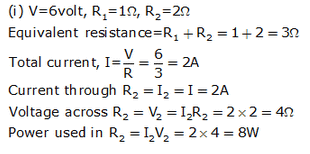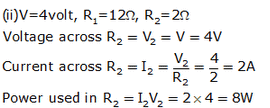Question 24:
Two lamps, one rated 40 W at 220 V and the other 60 W at 220 V, are connected in parallel to the electric supply at 220 V.
(a) Draw a circuit diagram to show the connections.
(b) Calculate the current drawn from the electric supply.
(c) Calculate the total energy consumed by the two lamps together when they operate for one hour.

Solution : Given 2 lamps:P1 = 40W. P2 = 60W
V = 220V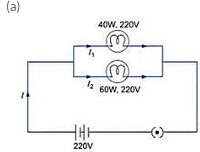(b) Voltage across both the bulbs is same and is equal to 220V.
Current through 40W lamp = I1 = P1/N = 40/220 A
Current through 60W lamp =I2 = P2N = 60/220 A
Total current drawn from the electric supply = 40/220 + 60/220 = 0.45 A

(a) Energy consumed by 40 W lamp in 1 hr. E1 = P1 x t = 40 x 1 = 40Wh
1Wh = 3.6 kJ
E1 = 40 x 3.6 = 144KJ
Energy consumed by 60W lamp in 1 hr. E2 = P2 x t = 60 x 1= 60Wh = 216 kJ
Total energy consumed = 144 + 216 = 360 kJ

Question 25:
An electric kettle connected to the 230 V mains supply draws a current of 10 A. Calculate :
(a) the power of the kettle.
(b) the energy transferred in 1 minute.

Solution :

Given V = 230V, I = 10amp

(a) P = VI

P = 230X10

P = 2300watt = 2300 Hs

(b) Energy consumed in minute = P x t = 23001/s x 60s = 138000J

Question 26:
A 2 kW heater, a 200 W TV and three 100 W lamps are all switched on from 6m. to 10 p.m. What is the total cost at Rs. 5.50 per kWh ?

Solution :

For heater:
P = 2kW, t = 4h
E = Pxt = 2x4 = 8kWh
For TV:
P = 200W = 0.2kW, t = 4h
E = Pxt = 0.2x4 = 0.8kWh
Lamps:
P = 100W = 0.1kW, t = 4h, n = 3
E = nxPxt = 3x0.1x4 = 1.2kWh
Total energy consumed = 8+0.8+1.2 = 10kWh
Cost of 1kWh = Rs. 5.50
Cost of 10kWh = Rs. 5.50 x 10 = Rs. 55

Question 27:
What is the maximum power in kilowatts of the appliance that can be connected safely to a 13 A ; 230 V mains socket ?

Solution :

I = 13amp, V = 230V

Power = VI

=230X13

= 2990W

P = 2.99kW

Question 28:
An electric fan runs from the 230 V mains. The current flowing through it is 0.4 A. At what rate is electrical energy transferred by the fan ?

Solution : Given :- V = 230V. I = 0.4amp

Rate at which electric energy is transferred = Power

Power = Vxl

= 230 x 0.4

= 92 W

= 92 J/s

Question 29:
(a) What is meant by “electric power” ? Write the formula for electric power in terms of potential difference and current.
(b) The diagram below shows a circuit containing a lamp L, a voltmeter and an ammeter. The voltmeter reading is 3 V and the ammeter reading is 0.5 A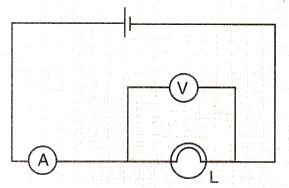(i) What is the resistance of the lamp ?
(ii) What is the power of the lamp ?
(c) Define kilowatt-hour. How many joules are there in one kilowatt-hour ?
(d) Calculate the cost of operating a heater of 500 W for 20 hours at the rate of ? Rs 3.90 per unit.

Solution :

(a) The rate at which electrical work is done or the rate at which electrical energy is consumed, is known as electric power.

It is given by

P = VI = watt

(b) Given: V = 3V, I = 0.5amp

(i) R = ?

We know that V = IR

3 = 0.5R

R = 6ohms

(ii) Power of lamp-VI

= 3x05

= 1.5watt

(c) One kilowatt hour is the amount of electrical energy consumed when an electrical appliance having a power rating of 1 kilowatt is used for 1 hour.

1kWh = 3.6x 1061

(d) Given P = 500W = 0.5kW. t = 20hr

We know that

Energy consumed = Pxt = 0.5X20

= 10kwh

Total cost = 10xcost per uint

Cost per unit = Rs. 3.9 per unit

Therefore. total cost = 10x3.9 = Rs 39

Page No:60

Question 41:
State whether an electric heater will consume more electrical energy or less electrical energy per second when the length of its heating element is reduced. Give reasons for your answer.
Solution :
By reducing the length of element the resistance will decrease.
Power is inversely proportional to resistance. So, this will result in more consumption of energy.

Question 42:
The table below shows the current in three different electrical appliances when connected to the 240 V mains supply :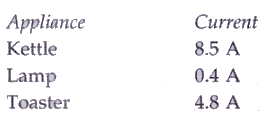(a) Which appliance has the greatest electrical resistance ? How does the data show this ?
(b) The lamp is connected to the mains supply by using a thin, twin-cored cable consisting of live and neutral wires. State two reasons why this cable should not be used for connecting the kettle to the mains supply.
(c) Calculate the power rating of the kettle when it is operated from the 240 V mains supply.
(d) A man takes the kettle abroad where the mains supply is 120 V. What is the current in the kettle when it is operated from the 120 V supply ?

Solution :
(a) Lamp; because least current is flowing through it.
(b) Large current drawn by the kettle; Earth connection needed.
(c) We know that
P = VI
V= 240V, I = 8.5A
P = 240X8.5 = 2040W = 2.04kW
(d) When connected to 240 V supply, P = 2040W
R = V2/P = 2402/240
R = 28.23ohm
Now, when V = 120V, R = 28.23ohm
I = V/R = 120/28.23 = 4.25A

Question 43:
A boy noted the readings on his home’s electricity meter on Sunday at 8 AM and again on Monday at 8 AM (see figure below).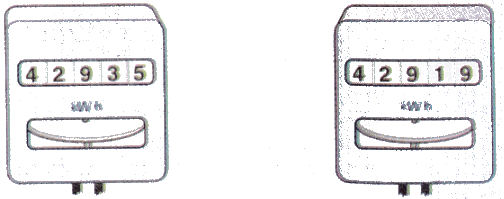(a) What was the meter reading on Sunday ?
(b) What was the meter reading on Monday ?
(c) How many units of electricity have been used ?
(d) In how much time these units have been used ?
(e) If the rate is Rs. 5 per unit, what is the cost of electricity used during this time ?

Solution :
(a) 42919
(b) 42935
(c) 42935-42919=16 units
(d) 24 hours
(e) Cost of 1 unit = Rs. 5
Cost of 16 units = 16×5 = Rs. 80

Question 44:
An electric bulb is rated as 10 W, 220 V. How many of these bulbs can be connected in parallel across the two wires of 220 V supply line if the maximum current which can be drawn is 5 A ?

Solution :
P = 10W, V = 220V, I = 5A
We know that
P = VI
= 220X5
P = 1100W
Power of one bulb = 10W
Total no. of bulbs that can be connected = 1100/10 = 110

Question 45:
Two exactly similar electric lamps are arranged (i) in parallel, and (ii) in series. If the parallel and series combination of lamps are connected to 220 V supply line one by one, what will be the ratio of electric power consumed by them ?

Solution :

Let resistance of each lamp = R ohms.
Case 1: Parallel connection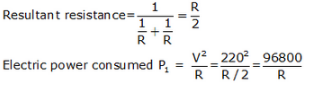Case 2: Series connection
Resultant resistance = R+R = 2R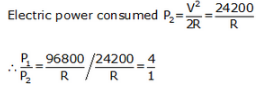Page No:66

Question 1:
How does the heat H produced by a current passing through a fixed resistance wire depend on the magnitude of current I ?
Solution :
Heat produced is directly proportional to the square of current.

Question 2:
If the current passing through a conductor is doubled, what will be the change in heat produced ?

Solution :
Heat produced is directly proportional to the square of current.
If current I is doubled, heat H will be four times.

Question 3:
Name two effects produced by electric current.

Solution :
Two effects of produced by electric current are:
(a) Heating effect
(b) Magnectic effect

Question 4:
Which effect of current is utilised in an electric light bulb ?

Solution :
Heating effect

Question 5:
Which effect of current is utilised in the working of an electric fuse ?

Solution :
Heating effect

Question 6:
Name two devices which work on the heating effect of electric current.

Solution :
Electirc heater and electric fuse.

Question 7:
Name two gases which are filled in filament type electric light bulbs.

Solution :
Argon and nitrogen.

Question 8:
Explain why, filament type electric bulbs are not power efficient.

Solution :
Filament type electric bulbs are not power efficien because most of the electric power consumed by the filament of a bulb appears as heat and only a small amount of electric power is converted into light.

Question 9:
Why does the connecting cord of an electric heater not glow hot while the heating element does ?

Solution :
The connecting cord of the heater made of copper does not glow because negligible heat is produced in it by passing current (because of its extremely low resistance); but the heating element made of nichrome glows because it becomes red-hot due to the large amount of heat produced on passing current (because of its high resistance).

Question 10:
(a) Write down the formula for the heat produced when a current I is passed through a resistor R for time
(b) An electric iron of resistance 20 ohms draws a current of 5 amperes. Calculate the heat produced in 30 seconds.

Solution :

(a) Heat produced. H = I2Rt
(b) Given: R = 20ohm, I = Samp, t = 30s
We know that H = 12Rt
H = 52x20x30
H = 150001

Question 11:
State three factors on which the heat produced by an electric current depends. How does it depend on these factors ?

Solution :
Heat produced by an electric current depends on the following factors:
(i) Heat produced is directly proportional to square of current.
(ii) Heat produced is directly proportional to resistance.
(iii) Heat produced is directly proportional to the time for which current flows.

Question 12:
(a) State and explain Joule’s law of heating.
(b) A resistance of 40 ohms and one of 60 ohms are arranged in series across 220 volt supply. Find the heat in joules produced by this combination of resistances in half a minute.

Solution :

(a) Joules law of heating states that heat produced injou les when a current of I amperes flows in a wire of resistance R ohms for time t seconds is
given by H = I2Rt.
Thus the heat produced In a wire Is directly proportional to:
(i) Square of current
(ii) Resistance of wire
(iii) Time for which current is passed
(b) Given: R1= 40ohms. R= 60ohms lin seriesI.V = 220V. t = 30sec
We know that
Total resistance. R = 40+60 = 100ohms
By Ohm's law,
V = IR
I = V/R
I = 220/100 = 2.2amp
Putting the values of I, R and t in eq. H = I2RT
H = 2.22 X 100 X 30
H =14520 J

Question 13:
Why is an electric light bulb not filled with air ? Explain why argon or nitrogen is filled in an electric bulb.

Solution :
If air is filled in an electric bulb, then the extremely hot tungsten filament would burn up quickly in the oxygen of air. So, the electric bulb is filled with a chemically unreactive gas like argon or nitrogen. Thes gases do not react with the hot tungsten filament and hence prolong the life of the filament of the bulb.

Question 14:
Explain why, tungsten is used for making the filaments of electric bulbs.

Solution :
Tungsten is used for making the filaments of electric bulbs because it has a very high melting point. Due to its very high melting point, the tungsten filament can be kept white hot without melting away. Also, tungsten has high flexibility and low rate of evaporation at high temperature.

Question 15:
Explain why, the current that makes the heater element very hot, only slightly warms the connecting wires leading to the heater.

Solution :
The connecting wires of the heater get only slightly warm because they have extremely low resistance due to which negligible heat is produced in them by passing current.

Question 16:
When a current of 4.0 A passes through a certain resistor for 10 minutes, 2.88 x 104 J of heat are produced. Calculate :
(a) the power of the resistor.
(b) the voltage across the resistor.

Solution : Given: I = 4amp. t = 10min = 10x60 = 600sec. H = 2.88x104.J

(a) We have

H = I2RT

28800=42xRx600

R-3ohms

We know that

P = 12xR

= 42x3

P = 48W

(b) V=?

We know that

V = IR

V = 4x3

V = 12V

Question 17:
A heating coil has a resistance of 200 Ω. At what rate will heat be produced in it when a current of 2.5 A flows through it ?

Solution :

Given: R = 200ohms, I = 2.5amp.t = lsec
We know that
H = I2RT
H = 2.52X200X1
H = 1250 J/s

Question 18: An electric heater of resistance 8Ω, takes a current of 15 A from the mains supply line. Calculate the rate at which heat is developed in the heater.

Solution :

Given: R=8ohms. I = 15amp. t = 1sec

We know that
H =12RT
H = 152X8X1
H = 1800J/s

Question 19:
A resistance of 25 Ω is connected to a 12 V battery. Calculate the heat energy in joules generated per minute.

Solution : Given: R = 25ohms. V = 12V. H = ?. t = 60sec

V= I R
12=25X1
I = 0.48amp
We have
H = I2RT
H = 0.482X25X60
H = 345.6J

Question 20:
100 joules of heat is produced per second in a 4 ohm resistor. What is the potential difference across the resistor ?

Solution : Given:H = 100J, t = 1sec, R = 4ohms.

We know that

H=I2RT

100 = I2X4X1

100/4 = I2

I = 5amp

V = IR

V = 5X4

= 20V

Question 21:
(a) Derive the expression for the heat produced due to a current ‘I’ flowing for a time interval ‘t’ through a resistor ‘R’ having a potential difference ‘V’ across its ends. With which name is this relation known ?
(b) How much heat will an instrument of 12 W produce in one minute if it is connected to a battery of 12 V ?
(c) The current passing through a room heater has been halved. What will happen to the heat produced by it ?
(d) What is meant by the heating effect of current ? Give two applications of the heating effect of current.
(e) Name the material which is used for making the filaments of an electric bulb.

Solution :

(a) When an electric charge Q moves against a p.d. V, the amount of work done is givenn by

W = Qx V ---------(1)
We know, current, I = Q/t
Q = I x t -----(2)
By Ohm's law, — V/I = R
V = IxR  (3)
Putting eqs. (2) and (3) in eq, (1),
W = I x t x 1x R
W = I2Rt

Assuming that all the electrical work done is converted into heat energy, we get

Heat produced, H = I2Rt joules

This relation is known as Joule's law of heating.

(b) Given:P = 12W. V = 12V. t = 60sec

Pr = VI

I = P/V = 12/12 = 1A

V = IR

R = V/I = 12/1 = 12ohm

H = 12Rt

H = 12x12x60

H = 720J

(c) The heat produced by the heater will become one-fourth because heat produced is cirectly proportional to the square of the current.

(d) When an electric current is passed through a high resistance wire. the wire becomes very hot and produces heat. This effect is knows as heating effect of current. This effect is used in room heaters and electric ovens.

(e) Tungsten is used for making the filaments of an electric bulb.

Page No:67

Question 31:
The electrical resistivities of four materials P, Q, R and S are given below :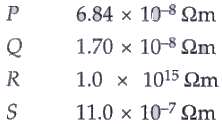Which material will you use for making : (a) heating element of electric iron (b) connecting wires of electric iron (c) covering of connecting wires ? Give reason for your choice in each case.
Solution :
(a) S; because it has high resistivity of 11/10000000 ohm-m (it is actually nichrome).
(b) Q; because it has very low resistivity of 1.7/100000000 ohm-m (it is actually copper).
(c) R; because it has very very high resistivity of 1.0X100000000000000 ohm-m (it is actually rubber).

Question 32:
(a) How does the wire in the filament of a light bulb behave differently to the other wires in the circuit when the current flows ?
(b) What property of the filament wire accounts for this difference ?

Solution :
(a) The filament wire becomes white hot where as other wires in the circuit do not get heated much.
(b) High resistance of filament wire accounts for this difference.

Question 33:
Two exactly similar heating resistances are connected (i) in series, and (ii) in parallel, in two different circuits, one by one. If the same current is passed through both the combinations, is more heat obtained per minute when they are connected in series or when they are connected in parallel ? Give reason for your answer.

Solution :
In series, because total resistance in series connection is more than that in parallel connection.

Question 34:
An electric iron is connected to the mains power supply of 220 V. When the electric iron is adjusted at ‘minimum heating’ it consumes a power of 360 W but at ‘maximum heating’ it takes a power of 840 W. Calculate the current and resistance in each case.

Solution :

Given: V = 220V. Pmin=360W. Pmax = 840W
For minimum heating case:
We know that
Pmin = VI
360 = 220X1
I = 1.63amp
R = V/I
R = 22011.63
R =134.96ohms

For maximum heating case:
We know that
Pmax = VI
840 = 220X1
I = 3.81amp
R = V/I
R = 220/3.81
R = 57.74ohms

Question 35:
Which electric heating devices in your home do you think have resistors which control the flow of electricity ?

Solution :
Electric iron , electric oven, water heater, room heater.

Offer running on EduRev: Apply code STAYHOME200 to get INR 200 off on our premium plan EduRev Infinity!

61 docs

,

,

,

,

,

,

,

,

,

,

,

,

,

,

,

,

,

,

,

,

,

,

,

,

;